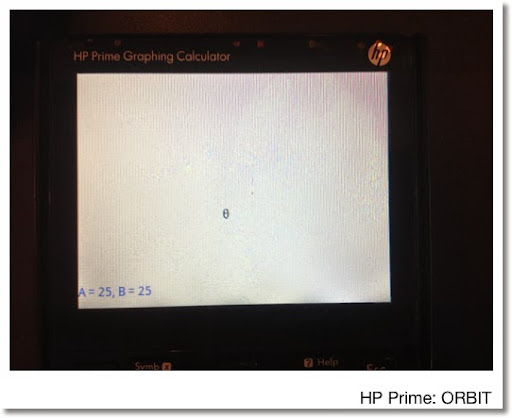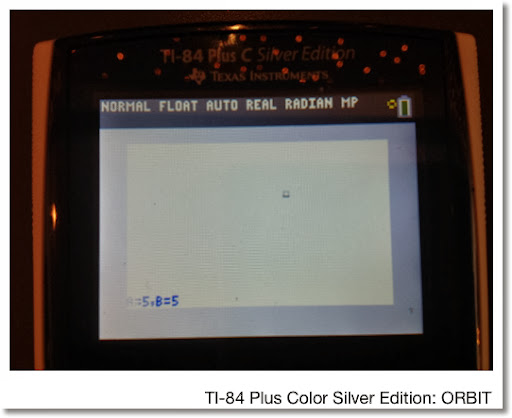## Saturday, March 1, 2014

### HP Prime/TI 84 Plus Color Silver Edition: Dynamic Programming: Orbit on a elliptic path.

This program orbits a θ character on a elliptical path. Use the arrow keys to change the size and the elliptical path. The program executes until ENTER is pressed.

Controls:
Left: Decrease of horizontal semi-axis
Right: Increase of horizontal semi-axis
Up: Increase of vertical semi-axis
Down: Decrease of vertical semi-axis
ENTER: Exits the program

HP Prime
EXPORT ORBIT( )
BEGIN
LOCAL A,B,K,I,X,Y;

// Initialize
A:=25; B:=25; I:=0;
X:=160+A*COS(I);
Y:=120-B*SIN(I);
MSGBOX("Press Enter to exit.");
RECT( );

REPEAT
// clear old placements and text
RECT_P(X,Y,X+10,Y+12);
RECT_P(0,218,319,239);

// get input from keyboard
K:=GETKEY;

IF K==7 AND A>1 THEN
A:=A+1; END;
IF K==8 AND A<50 THEN
A:=A-1; END;
IF K==2 AND B<50 THEN
B:=B+1; END;
IF K==12 AND B>1 THEN
B:=B-1; END;

// Move the satellite
I:=I+π/64;
X:=160+A*COS(I);
Y:=120-B*SIN(I);
TEXTOUT_P("θ",X,Y,2);
TEXTOUT_P("A = "+STRING(A)+", B = "+STRING(B),
0,2,#FFh);
WAIT(0.05);
UNTIL K==30;
END;

TI-84 Color Plus Silver Edition
Note: Pixels on the newer Color edition (size 250 × 150 approximately for the graph screen) opposed to the classic black and white TI-84+ (62 × 94)

Program: ORBIT
: ZStandard
: ZSquare
: FnOff
: 5 → A
: 5 → B
: 0 → I
: ClrDraw
: AxesOff
: Repeat K=105
: Pt-Off(A cos(I), B sin(I), 2
: Text(150,0," " // 32 spaces - there is no box command
: GETKEY→ K
: If K=24 and A>1
: A-1 → A
: If K=26 and A<10
: A+1→ A
: If K=25 and B<10
: B+1→ B
: If K=34 and B>1
: B-1 → B
: I + π/16 → I
: Pt-On(A cos(I), B sin(I), 2
: Text(150,0,"A =",A,",B =",B
: End
: AxesOnThis blog is property of Edward Shore. 2014

### Retro Review: Radio Shack EC-4026

Retro Review:   Radio Shack EC-4026 Quick Facts: Model:  EC-4026 Company:  Radio Shack Equivalent Of:  Casio fx-4500P, Casio fx-4500PA Years...